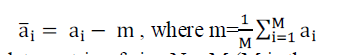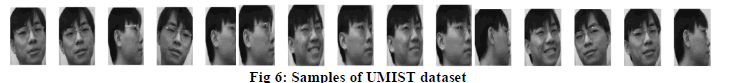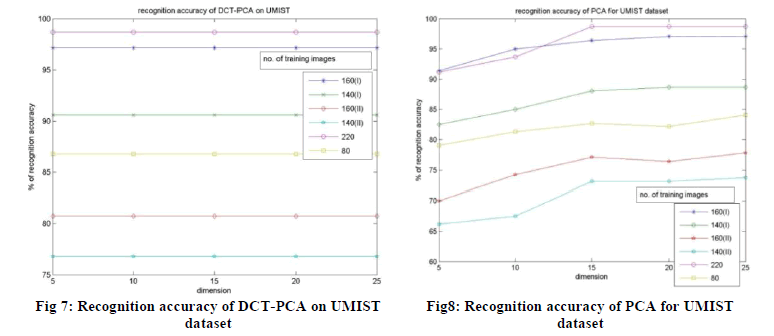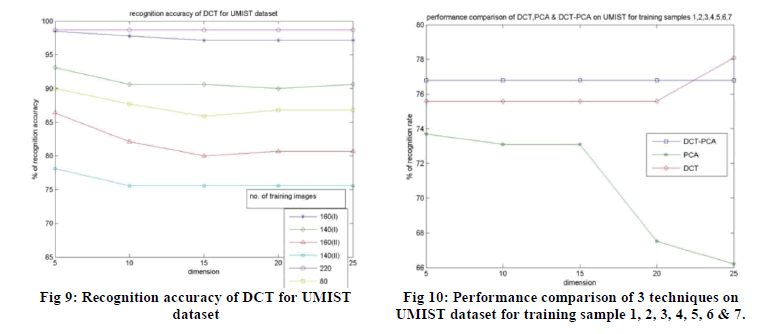ISSN ONLINE(2320-9801) PRINT (2320-9798)

All submissions of the EM system will be redirected to Online Manuscript Submission System. Authors are requested to submit articles directly to Online Manuscript Submission System of respective journal.

# Discriminative DCT Based Face Recognition: An Efficient and Accurate Approach

 M Sharmila Kumari, Swathi Salian Department of Computer Science and Engineering, P A College of Engineering, Nadupadavu, Mangalore, India Related article at Pubmed, Scholar Google

Visit for more related articles at International Journal of Innovative Research in Computer and Communication Engineering

## Abstract

In this paper, we study an efficient face recognition technique using combined approaches of discrete cosine transform (DCT) and principal component analysis (PCA). In DCT-PCA, PCA is applied onto the extracted DCT coefficients of the face images. This technique is based on the Fourier spectra of the facial images. The experiments are conducted on the standard face datasets such as ORL, UMIST and real dataset and the performance of the DCT-PCA technique is compared with the existing DCT and PCA. From the experimental results, it is proved that the DCT-PCA based technique for face recognition has improved recognition rate than the conventional PCA and DCT approaches.

### Keywords

Discrete Cosine Transform, Principal Component Analysis, Face recognition.

### INTRODUCTION

Biometric based techniques have gained popularity in recent years. It has become the successful option for identifying the individuals. Any physiological/behavioral characteristics can be used to identify an individual as long as it is permanent (invariant) or distinct for each individual . The face recognition techniques have become an active research area in recent years. Face recognition is a technique to recognize the person face from a still image or anyvideo. To develop such a system, we use image database of face images .
Several approaches for face recognition have been presented in the literature. Dimensionality reduction is essential for extracting effective features and reducing computational complexity in classification stage. Principal component analysis (PCA) , , linear discriminate analysis (LDA)  and discrete cosine transform (DCT)  are the main techniques used for data reduction and feature extraction in the appearance based approaches. The PCA based models operate in vectors. They use Eigenface based approaches. In the literature of pattern recognition, several PCA variants are in use such as modular PCA , Kernel PCA , independent component analysis  etc. The two dimensional (2D) PCA eliminates the need of converting 2D image matrices into 1D vector unlike conventional PCA . While the 2D PCA worked only in one direction, either in row or column, two directional 2D PCA work in both directions simultaneously . On the other hand, DCT based techniques extracts most significant DCT coefficients of the training images as a part of feature extraction. Certain normalization techniques can improve the robustness of the DCT based face recognition system to variations in facial geometry and illumination . The DCT can either be applied to entire frontal face image to obtain global DCT features or the local features like nose, eyes etc can be extracted manually and then DCT can be applied onto them to form local DCT features. When local and global features are combined, DCT gives a relatively high recognition rate . In DCT-PCA based face recognition technique, PCA is directly applied on the extracted DCT coefficients of the face images, thus achieving dimensionality reduction and also improved recognition rates .
In this paper, we have made an attempt to study the DCT-PCA based technique for face recognition. We also compare this technique with the conventional PCA and DCT and present a comparative study. The experimentations are performed on the standard face datasets like ORL, UMIST and a real dataset. The rest of the paper is organized as follows: PCA is discussed in section 2, DCT and DCT-PCA based face recognitions are discussed in section 3, the section 4 presents the experimental results. The final work is concluded in section 5.

### PRICIPAL COMPONENT ANALYSIS BASED FACE RECOGNITION

The idea of using PCA as feature extraction technique to represent face images was first developed by Kirby and Sirovich . This approach was later used in face representation and recognition technique by Turk and Pentland . The PCA used Eigenface based technique, where in the 2D image matrices of all the training face images are transformed into 1D vectors and then represented as a point in the high dimensional vector space, known as Eigen vector space.
Let a1,a2,a3,….,aMbe M training samples each of size r x c. Each 2D image matrix ai (i=1,2,…,M) is converted into 1D image vector and then mean centered by subtracting the mean image from each vector as follows:(1)
The data matrix of size N x M (M is the number of training images& N=r*c, is the number of elements in ai) is formed by using the mean centered image vectors as:(2)
Then we compute covariance matrix by multiplying data matrixand its transpose:(3)
The Eigen vectors of the covariance matrix are computed and kEigen vectors corresponding to the klargest Eigen values are ordered in descending order to form the Eigenface space. Let, be obtained Eigenface space.
Once Eigen space is obtained, each of the centered training image vectors (ai) are projected into the Eigen space.(4)
In the classification stage, first test image is mean centered by subtracting the mean image from the test image vector. It is then projected into the obtained Eigen vector space as:(7)
And(6)
The comparison is done between the projected test image and each of the training images using similarity measure. The training image found to be closest to the test image is used to classify the test image. The most well known similarity measure is Euclidean distance.

### DCT AND DCT-PCA BASED APPROACHES

The DCT is an invertible linear transform that can express a finite sequence of data points in terms of a sum of cosine functions oscillating at different frequencies. The original face image is converted to frequency domain by applying 2D DCT. The original image can be restored back from the DCT coefficients by using invert 2D DCT .
In DCT based face recognition, important frequency components of the training image, obtained by DCT function is extracted. The feature vectors for the training images contain this extracted, more relevant information. In the classification stage, the feature vectors obtained for the training images and test image are compared using a similarity measure, mostly Euclidean distance. A training image with minimum distance is used to classify the test image. Given an r by c image f(r,c), 2D r by c DCT is defined as follows:In DCT-PCA based approach, initially the 2D DCT function given in equation (7) is applied on the face images to obtain the DCT coefficients. Among these coefficients, we extract only those coefficients that represent more relevant information. Once the relevant frequency components are extracted, PCA is then applied onto them for feature extraction . By applying PCA on the extracted DCT coefficients, higher compression rate is achieved and also recognition rate can be improved.

### EXPERIMENTAL RESULTS

Our experimentation involves use of two standard face datasets ORL and UMIST and a real dataset, which consists of faces with slight variations in pose, illumination, expressions etc.

#### Results on ORL face dataset

The ORL dataset consists ofgrayscale face images of 40 persons with 10 samples of each. The images are rescaled to 112 x 92 sizes. They represent slight variations in pose and expressions. The fig.1 shows the sample images of ORL dataset.The experimentation includes varying the number of training samples in various ways. We have considered first alternate samples such as (i) 1, 3, 5, 7, 9, (ii) 2, 4, 6, 8, 10 for training and rest of the samples for testing. Then we also considered for training, the continuous samples (iii) 1, 2, 3, 4, 5, (iv) 6, 7, 8, 9, 10, (v) random 7 and (vi) random 4 samples of all face images for training and remaining for testing. In all the above experiments, we record the recognition accuracy of the implemented algorithm under different dimensions of feature vector. The different dimensions considered in our work are 5, 10, 15, 20 and 25.
In this paper, we have considered DCT-PCA for implementation and its performance is compared with traditional PCA and DCT. The experimental results obtained using DCT-PCA on ORL dataset is plotted in the fig 2. In DCT-PCA, first, we applied 2D DCT function on the training face images; from the DCT coefficients of the face images, 100 most relevant frequency components are extracted. On these extracted DCT coefficients of the face images, PCA is applied, which further reduces the dimensionality of the features extracted from the images. In order to perform a comparative analysis, we have conducted the same set of experiments mentioned above on PCA and DCT. The results obtained by using PCA on ORL dataset is shown in Fig. 3.The performance of DCT technique on ORL dataset is shown in fig. 4. From the experimental results obtained on ORL dataset, we can say that the performances of DCT and DCT-PCA are better than PCA. Also the DCT-PCA performs better than DCT in most cases, except when there is small number of training samples. And also, the number of coefficients for face representation used by DCT-PCA is less compared to DCT. Therefore, we can say that the DCTPCA outperforms the other two approaches, which is clearly shown in fig. 5 that plots the performances of 3 algorithms on ORL for the training samples 6, 7, 8, 9 and 10.#### Results on UMIST face dataset

Another dataset considered for experimentation in this paper is UMIST dataset. It consists of face images of 20 persons with varying number of samples of each. For our experiments, we have considered only 15 samples of each person. The fig.6 shows 15 such samples of a person in UMIST.The same set of experiments that are conducted on ORL, are also performed on UMIST dataset for all the different face recognition approaches mentioned in this paper. That is, here out of 15 samples of each person, we have considered subset of samples for training and rest for testing in the similar way as in ORL. The recognition rates obtained using DCT-PCA, PCA and DCT on UMIST are plotted in fig. 7, fig. 8 and fig. 9 respectively.
From the experimental results obtained on UMIST dataset, we can analyze that the DCT and DCT-PCA outperforms the conventional PCA as in the case of ORL dataset. On the other hand, the DCT and DCT-PCA performs almost in the similar manner on UMIST dataset, in some cases DCT-PCA performs better. Whereas the performance of DCT improves even when there is decrease in dimension of feature vector. Since the DCT-PCA uses less number of coefficients for face representation than DCT, we can say that DCT-PCA is an efficient face recognition technique. The fig. 10 shows a plot of the performances of 3 algorithms on UMIST for the training samples 1, 2, 3, 4, 5, 6 and 7, which clearly shows that the DCT-PCA performs better.#### Results on real face dataset

We also have extended our work on real face dataset, which consists of 16 persons each with 7 face samples. They represent slight variations in pose, expression and illumination. The 7 such samples of a person in real dataset are shown in fig. 11.The experimental results obtained using DCT-PCA, PCA and DCT on real dataset are shown below in the Fig. 12, 13 and 14 respectively. We have kept almost the same experimental set up that are used on ORL and UMIST for real dataset as well, except thatonly 1 set of random samples (random 5 samples 1,3,4,6,7) is considered for training, unlike the case of ORL or UMIST.In the case of real dataset, the DCT-PCA doesn’t produce an improved recognition rate than DCT, but gives a better result than PCA. But we can still say that among three approaches, DCT-PCA is better since it uses very less amount of coefficients for face representation and achieves higher compression than DCT. As in the case of ORL and UMIST, the performance of DCT-PCA does not go down with decrease in the dimension of feature vector even in real dataset, which is an advantage.### CONCLUSION

In this paper, we implemented and tested the three approaches for face recognition, PCA, DCT and an integrated approach called as DCT-PCA. The experiments are carried out on the standard datasets like ORL and UMIST and a real dataset. Various experiments are conducted using the three algorithms on these datasets; it included varying the number of training samples in different possible ways under different dimensions of feature vector.
From the experimental results, we conclude that the DCT and DCT-PCA techniques, which works in frequency domain, gives better recognition accuracy than PCA that work in spatial domain. Also, the DCT-PCA which is a combination of DCT and PCA, works better than other two techniques in terms of recognition accuracy and computationally efficiency.

### References

1. Jain et al., “An introduction to biometric recognition”, IEEE transactions on circuits and systems for video technology, vol. 14, no. 1, January 2004.

2. Jigar M. Pandya, DevangRathod, Jigna J. Jadav, “A Survey of Face Recognition approach “, International Journal of Engineering Research and Applications (IJERA) ISSN: 2248-9622 Vol. 3, Issue 1,, pp.632-635, January -February 2013.

3. M. Kirby and L. Sirovich,“Application of the Karhunen-Lokve procedure for the characterization of human faces”, IEEEtransactions on pattern analysis and machine intelligence. vol. 12.no. 1 . january 1990.

4. M. A. Turk and A. P. Pentland, “Eigenfaces for recognition,” J. Cogn.Neurosci., vol. 3, pp. 71–86, 1991.

5. C. Zhou, et al., “Face recognition based on PCA image reconstruction and LDA”, Optik - Int. J. Light Electron Opt., http://dx.doi.org/10.1016/j.ijleo.2013.04.108 (2013).

6. Prof. AnandNajan, Prof. Mrs. A. C. Phadke, “DCT Based Face Recognition”, International Journal of Engineering Innovation & Research, Volume 1, Issue 5, ISSN : 2277 – 5668.

7. RajkiranGottumukkal, VijayanK.Asari, “An improved face recognition technique based on modular PCA approach”, Pattern Recognition Letters25 ,429–436, (2004).

8. M.H. Yang, “Kernel Eigenfaces vs. Kernel Fisherfaces: Face Recognition Using Kernel Methods,” Proc. Fifth IEEE Int’l Conf. Automatic Face and Gesture Recognition (RGR’02), pp. 215-220, May 2002.

9. M.S. Bartlett, J.R. Movellan, and T.J. Sejnowski, “Face Recognition by Independent Component Analysis,” IEEE Trans. Neural Networks, vol.13, no. 6, pp. 1450-1464, 2002.

10. J. Yang, D. Zhang, A.F. Frangi, J.Y. Yang, “Two-dimensional PCA: a new approach to appearance based face representation and recognition”,IEEE Trans. Pattern Anal. Mach. Intell. 26 (1) 131–137, (2004).

11. Daoqiang Zhang, Zhi-Hua Zhou,“ (2D2 PCA): Two-directional two-dimensional PCA for efficient face representation and recognition”,Neurocomputing 69, 224–231,(2005).

12. Ziad m. Hafed and Martin D. Levine, “Face recognition using the discrete cosine transform”, International Journal of Computer Vision 43(3),167–188, 2001.

13. Aman R. Chadha, Pallavi P. Vaidya, M. Mani Roja, “Face Recognition Using Discrete Cosine Transform for Global and Local Features”, Proceedings of the 2011 International Conference on Recent Advancements in Electrical, Electronics and Control Engineering (IConRAEeCE) IEEE Xplore: CFP1153R-ART; ISBN: 978-1-4577-2149-6.

14. Swarup K.D, Sukadev Maher, “New Technique forDCT-PCA Based Face Recognition”, InternationalConference on ElectronicSystems,http://hdl.handle.net/2080/1356, 2011.